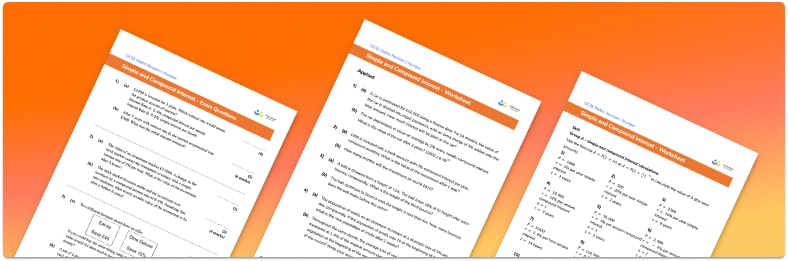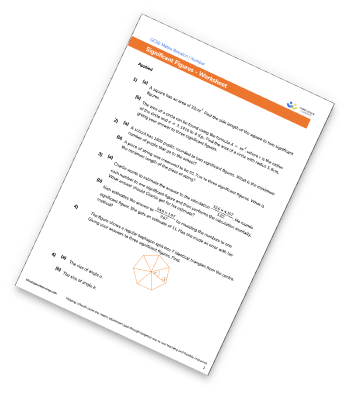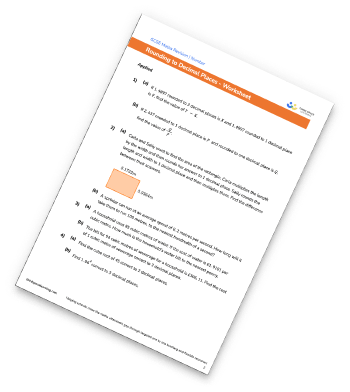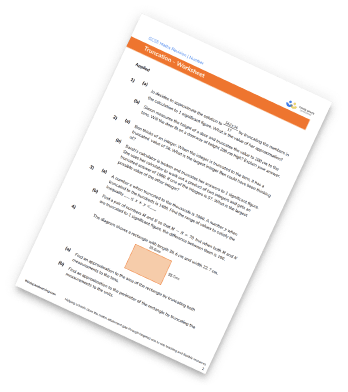# Simple And Compound Interest Worksheet• Section 1 of the simple and compound interest worksheet contains 36 skills-based simple and compound interest questions, in 3 groups to support differentiation
• Section 2 contains 4 applied simple and compound interest questions with a mix of worded problems and deeper problem solving questions
• Section 3 contains 4 foundation and higher level GCSE exam style simple and compound interest questions
• Answers and a mark scheme for all simple and compound interest questions are provided
• Questions follow variation theory with plenty of opportunities for students to work independently at their own level
• All questions created by fully qualified expert secondary maths teachers
• Suitable for GCSE maths revision for AQA, OCR and Edexcel exam boards
• This field is for validation purposes and should be left unchanged.

You can unsubscribe at any time (each email we send will contain an easy way to unsubscribe). To find out more about how we use your data, see our privacy policy.

### Simple and compound interest at a glance

For GCSE we need to know about two different types of interest – simple and compound.

Simple interest calculates interest based purely on the original amount invested. For example, a savings account with a simple interest rate of 2% and an initial amount of £100, would earn £2 interest each year regardless of the amount of money in the account at the end of each year.

With compound interest, the amount of money in the account at the start of each year is used when calculating interest. For example, if a bank account has an initial investment of £100, and the compound interest rate is 2% per annum (per year), at the end of the first year, the value of the investment will be identical to the simple interest, £102. However, the next year’s interest calculation will be 2% of £102, rather than 2% of the original amount invested. This process will continue for the number of years the money is invested. The total amount of interest is greater when using compound interest than when using simple interest.

The annual compound interest formula is initial amount annual interest rate number of years. Annual interest rate is written as a percentage multiplier, so an interest rate of 2% would be a multiplier of 1.02. If interest is calculated over a different time period, such as semiannually, the ‘number of years’ would change to ‘number of times interest is paid’.

Compound depreciation works in the same way as compound interest, with a slight adjustment to the multiplier.

Looking forward, students can then progress to additional number worksheets, for example aor aFor more teaching and learning support on Number our GCSE maths lessons provide step by step support for all GCSE maths concepts.

## Related worksheets

Significant Figures WorksheetRounding To The Nearest 10, 100, And 1000 WorksheetRounding Decimals WorksheetTruncation Worksheet## Do you have KS4 students who need more focused attention to succeed at GCSE?There will be students in your class who require individual attention to help them succeed in their maths GCSEs. In a class of 30, it’s not always easy to provide.

Help your students feel confident with exam-style questions and the strategies they’ll need to answer them correctly with our dedicated GCSE maths revision programme.

Lessons are selected to provide support where each student needs it most, and specially-trained GCSE maths tutors adapt the pitch and pace of each lesson. This ensures a personalised revision programme that raises grades and boosts confidence.

Find out more